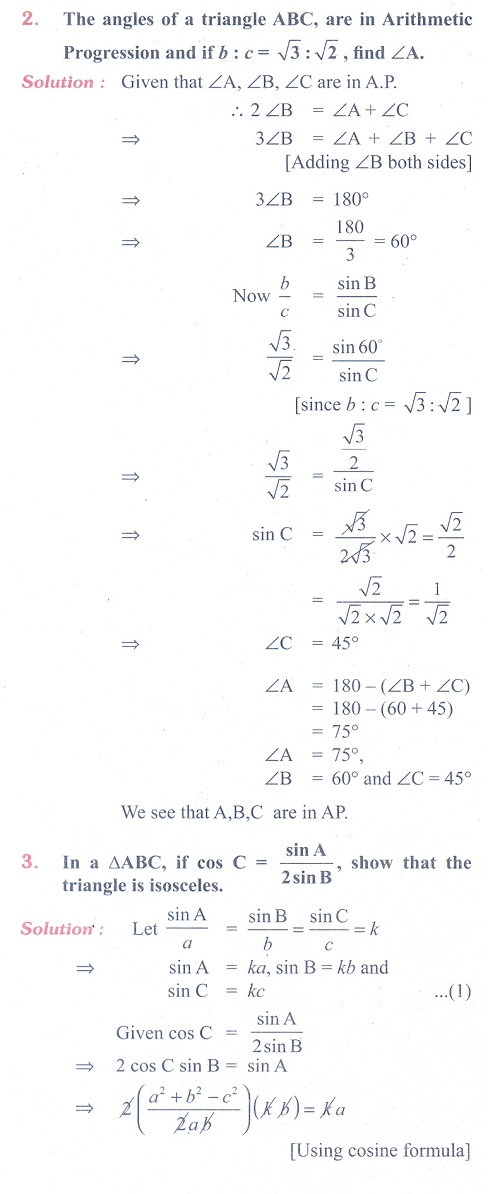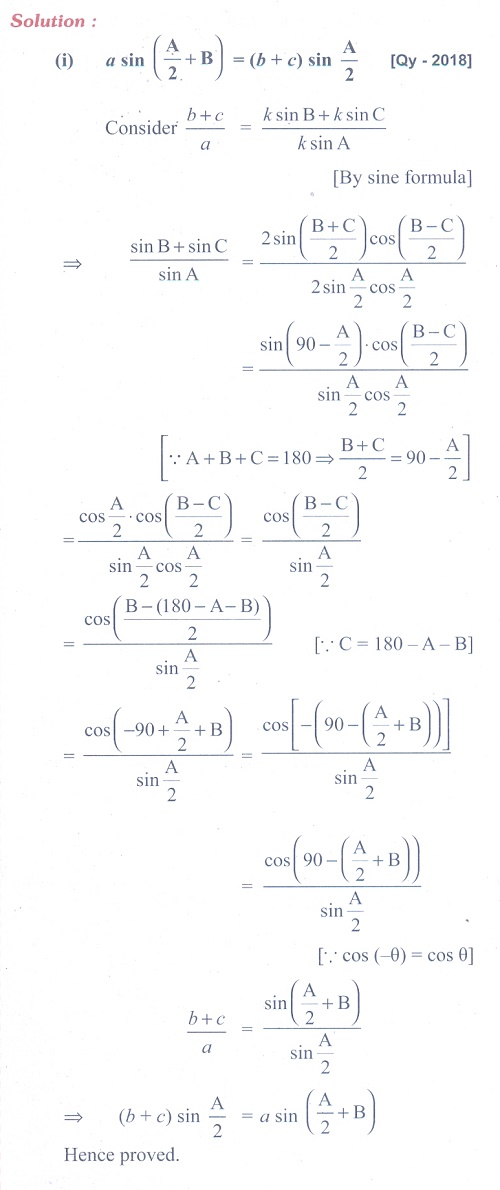Home | | Maths 11th std | Exercise 3.9: Area of a triangle (HeronŌĆÖs Formula)

# Exercise 3.9: Area of a triangle (HeronŌĆÖs Formula)

Maths Book back answers and solution for Exercise questions - Mathematics : Trigonometry: Area of a triangle (HeronŌĆÖs Formula)Tags : Problem Questions with Answer, Solution | Trigonometry | Mathematics , 11th Mathematics : UNIT 3 : Trigonometry
Study Material, Lecturing Notes, Assignment, Reference, Wiki description explanation, brief detail
11th Mathematics : UNIT 3 : Trigonometry : Exercise 3.9: Area of a triangle (HeronŌĆÖs Formula) | Problem Questions with Answer, Solution | Trigonometry | Mathematics